# Capillary waves

## Thermal capillary waves

Thermal capillary waves are oscillations of an interface which are thermal in origin. These take place at the molecular level, where only the surface tension contribution is relevant.

Capillary wave theory (CWT) is a classic account of how thermal fluctuations distort an interface (Ref. 1). It starts from some intrinsic surface that is distorted. By performing a Fourier analysis treatment, normal modes are easily found. Each contributes a energy proportional to the square of its amplitude; therefore, according to classical statistical mechanics, equipartition holds, and the mean energy of each mode will be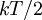$kT/2$. Surprisingly, this result leads to a divergent surface (the width of the interface is bound to diverge with its area) (Ref 2). This divergence is nevertheless very mild: even for displacements on the order of meters the deviation of the surface is comparable to the size of the molecules. Moreover, the introduction of an external field removes the divergence: the action of gravity is sufficient to keep the width fluctuation on the order of one molecular diameter for areas larger than about 1 mm2 (Ref. 2).

Recently, a procedure has been proposed to obtain a molecular intrinsic surface from simulation data (Ref. 3). The density profiles obtained from this surface are, in general, quite different from the usual mean density profiles.

## Gravity-capillary waves

These are ordinary waves excited in an interface, such as ripples on a water surface. Their dispersion relation reads, for waves on the interface between two fluids of infinite depth: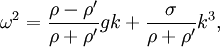$\omega^2=\frac{\rho-\rho'}{\rho+\rho'}gk+\frac{\sigma}{\rho+\rho'}k^3,$

where ω is the angular frequency, g the acceleration due to gravity, σ the surface tension, ρ and ρ‘ the mass density of the two fluids (ρ > ρ‘) and k the wavenumber.

### Derivation

This is a sketch of the derivation of the general dispersion relation, see Ref.  for a more detailed description.

Three contributions to the energy are involved: the surface tension, gravity, and hydrodynamics. The part due to gravity is the simplest: integrating the potential energy density due to gravity,$\rho g z$ from a reference height to the position of the surface,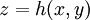$z=h(x,y)$: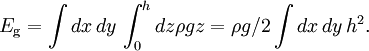$E_\mathrm{g}= \int dx\, dy\, \int_0^h dz \rho g z = \rho g /2 \int dx\, dy\, h^2.$

(For simplicity, we are neglecting the density of the fluid above, which is often acceptable.)

An increase in area of the surface causes a proportional increase of energy: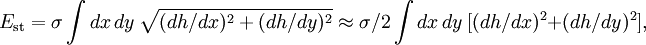$E_\mathrm{st}= \sigma \int dx\, dy\ \sqrt{(dh/dx)^2+(dh/dy)^2} \approx \sigma/2 \int dx\, dy\ [(dh/dx)^2+(dh/dy)^2],$

where the fist equality is the area in this (de Monge) representation, and the second applies for small values of the derivatives (surfaces not too rough).

The last contribution involves the kinetic energy of the fluid: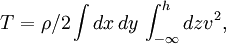$T= \rho/2 \int dx\, dy\, \int_{-\infty}^h dz v^2,$

where$v$ is the module of the velocity field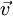$\vec{v}$. If the fluid is incompressible and its flow is irrotational (often, sensible approximations), its flow will be potential: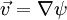$\vec{v}=\nabla\psi$, and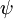$\psi$ must satisfy Laplace equation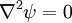$\nabla^2\psi=0$. This equation can be solved with the proper boundary conditions:$\vec{v}$ must vanish well below the surface (in the "deep water" case, which is the one we consider), and it vertical component must match the motion of the surface: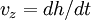$v_z=dh/dt$ at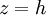$z=h$. Performing the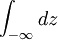$\int_{-\infty} dz$ integration one is left with a surface integral for the kinetic energy. One is then left with two contribution to the potential energy involving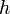$h$,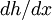$dh/dx$, and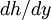$dh/dy$, and one for the kinetic energy involving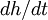$dh/dt$, all three being surface integrals. Constructing the Lagrangian of this system one readily finds a wave-like equation, whose oscillatory solutions satisfy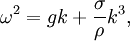$\omega^2=g k+\frac{\sigma}{\rho}k^3,$

the same dispersion as above if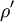$\rho'$ is neglected.